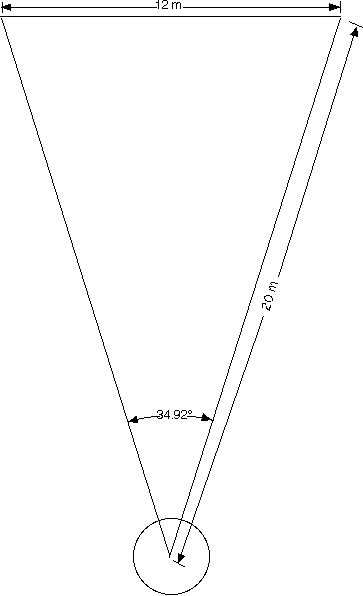Setting up a 34.92 degree sector

A 34.92 degree angle is the angle between the two equal sides of an isosceles triangle which has the unequal side. 0.6 times the length of the equal sides.  It can be mathematically expressed as 2arcsin(0.30).  Illustrated below is a typical layout for a shot circle.  Measure out from the center of the circle with two tapes.  Pull the two tapes tight and separate them by 12 meters at the 20 meter mark on each tape.  It will take 3 people and 3 tapes to do this.  One person holds the two tapes which will locate the sector lines in the center of the circle.  The second person pulls tight one of the sector line tapes and holds the zero mark of the cross measuring tape at the 20 meter mark.  The third person pulls tight the other sector line tape and holds the 12 meter mark of the cross measuring tape at the 20 meter mark.  The sector lines are centered on the stop board and permanent pins or stakes are placed at the ends of the sector lines.  The sector lines can be extended if longer throws than the ends of these sector lines
(18.93 m) are anticipated.

Sectors for the discus and hammer are set up in a similar manner, keeping the ratio of cross measurement length to sector line length at 0.6.  For example, one might use sector lines of 60 m and a cross measurement of 36 m for the discus and 70 m sector lines and a cross measurement of 42 m for the hammer.

Note that the javelin sector is set out in a similar manner, except the sector angle is 28.96 degrees, which is the angle between the two equal sides of an isosceles triangle having the unequal side 0.5 times the length of the equal sides and is mathematically expressed as 2arcsin(0.25).